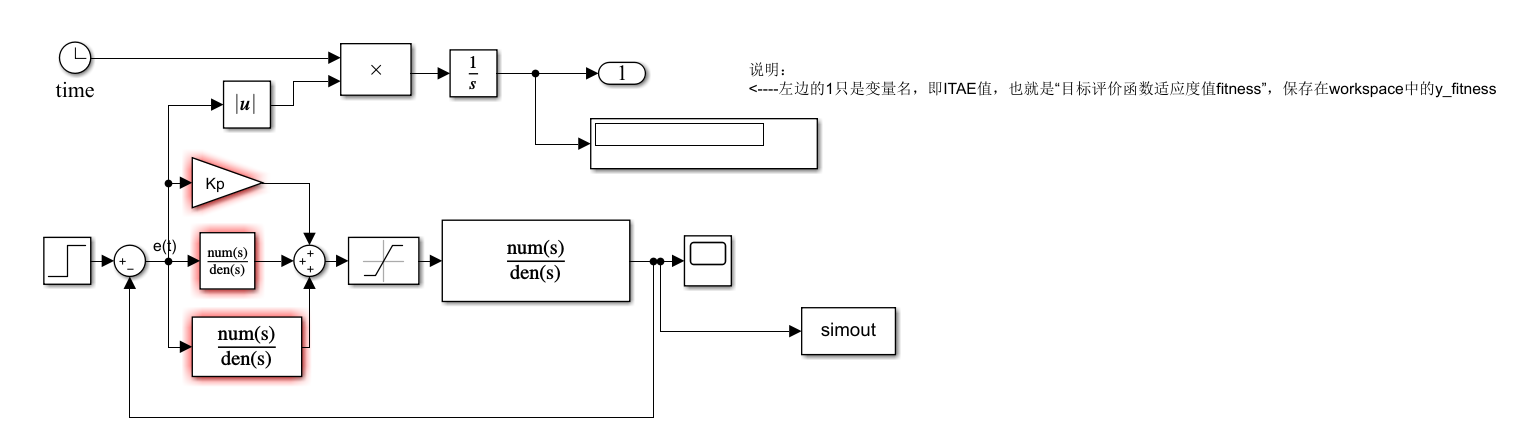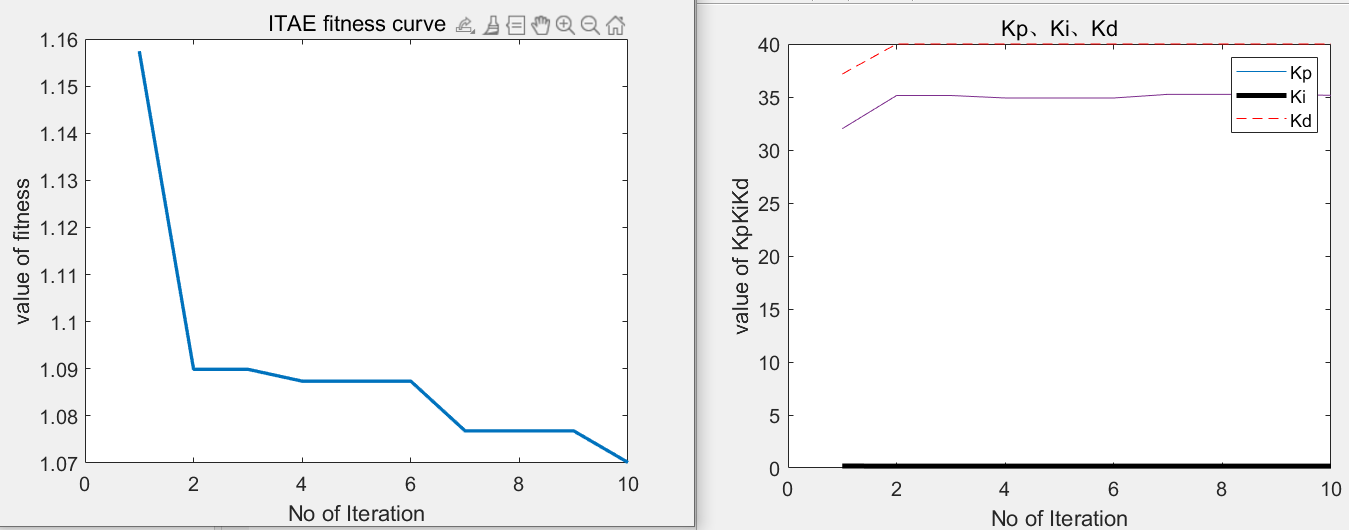• 粒子群算法优化PID参数实例
2022-03-18 18:23:55
%% 清空环境
clear all
clc
tic; %记录算法运行时间
%% 参数设置
%标准PSO算法
w = 0.6;      % 惯性因子
c1 = 2;       % 加速常数
c2 = 2;       % 加速常数

%%经验值
% w = 0.7298;      % 惯性因子
% c1 = 1.49618;       % 加速常数
% c2 = 1.49618;       % 加速常数

%%随机权重策略
% mean_max = 0.8;  %随机权重均值最大值
% mean_min = 0.5;  %随机权重均值最小值
% sigma = 0.2;          %方差

%以下参数值都可以改

Dim = 3;            % 维数
SwarmSize = 30;    % 粒子群规模
ObjFun = @PSO_PID;  % 待优化函数句柄，来自于PSO_PID.m

MaxIter = 10;      % 最大迭代次数
MinFit = 0.1;       % 最小适应值

Vmax = 1;
Vmin = -1;
% Ub = [300 300 300];  %变量上限
Ub = [40 1 40];
Lb = [0 0 0];        %变量下限

%% 粒子群初始化
Range = ones(SwarmSize,1)*(Ub-Lb);
Swarm = rand(SwarmSize,Dim).*Range + ones(SwarmSize,1)*Lb      % 初始化粒子群
VStep = rand(SwarmSize,Dim)*(Vmax-Vmin) + Vmin                 % 初始化速度
fSwarm = zeros(SwarmSize,1);
for i=1:SwarmSize
fSwarm(i,:) = feval(ObjFun,Swarm(i,:));                         % 粒子群的适应值
end

%% 个体极值和群体极值
[bestf, bestindex]=min(fSwarm);
zbest=Swarm(bestindex,:);   % 全局最佳
gbest=Swarm;                % 个体最佳
fgbest=fSwarm;              % 个体最佳适应值
fzbest=bestf;               % 全局最佳适应值

%% 迭代寻优
iter = 0;
y_fitness = zeros(1,MaxIter);   % 预先产生4个空矩阵
K_p = zeros(1,MaxIter);
K_i = zeros(1,MaxIter);
K_d = zeros(1,MaxIter);
while( (iter < MaxIter) && (fzbest > MinFit) )
for j=1:SwarmSize
%         miu = mean_min+(mean_max-mean_min)*rand;
%         w = miu +sigma*randn();

% 速度更新
VStep(j,:) = w*VStep(j,:) + c1*rand*(gbest(j,:) - Swarm(j,:)) + c2*rand*(zbest - Swarm(j,:));
if VStep(j,:)>Vmax, VStep(j,:)=Vmax; end
if VStep(j,:)<Vmin, VStep(j,:)=Vmin; end
% 位置更新
Swarm(j,:)=Swarm(j,:)+VStep(j,:);
for k=1:Dim
if Swarm(j,k)>Ub(k), Swarm(j,k)=Ub(k); end
if Swarm(j,k)<Lb(k), Swarm(j,k)=Lb(k); end
end
% 适应值
fSwarm(j,:) = feval(ObjFun,Swarm(j,:));
% 个体最优更新
if fSwarm(j) < fgbest(j)
gbest(j,:) = Swarm(j,:);
fgbest(j) = fSwarm(j);
end
% 群体最优更新
if fSwarm(j) < fzbest
zbest = Swarm(j,:);
fzbest = fSwarm(j);
end
end
iter = iter+1;                      % 迭代次数更新
y_fitness(1,iter) = fzbest;         % 为绘图做准备
K_p(1,iter) = zbest(1);
K_i(1,iter) = zbest(2);
K_d(1,iter) = zbest(3);
end

elapsed_time=toc % time taken for this algorithm

%% 绘图输出
figure(1)      % 绘制性能指标ITAE的变化曲线
plot(y_fitness,'LineWidth',2)
title('ITAE fitness curve','fontsize',12);
xlabel('No of Iteration','fontsize',12);ylabel('value of fitness','fontsize',12);
set(gca,'Fontsize',12);
%下面代码读取当前电脑时间命名并自动figure(1)保存为.fig格式，在当前文件位置
h1=figure(1);
fn1=['ITAE by PSO-',datestr(clock,'yy-mm-dd-HH-MM-SS'),'.fig'];
saveas(h1,fn1);

%下面图2是Kp Ki Kd的值变化过程，可以不画不保存
figure(2)      % 绘制PID控制器参数变化曲线
plot(K_p);
hold on
plot(K_i,'k','LineWidth',3);
plot(K_d,'--r');
title('Kp、Ki、Kd','fontsize',12);
xlabel('No of Iteration','fontsize',12);
ylabel('value of KpKiKd','fontsize',12);
set(gca,'Fontsize',12);
legend('Kp','Ki','Kd');
%下面代码读取当前电脑时间命名并自动figure(2)保存为.fig格式，在当前文件位置
h2=gcf;
fn2=['Kpid by PSO-',datestr(clock,'yy-mm-dd-HH-MM-SS'),'.fig'];
saveas(h2,fn2);矩阵
更多相关内容
• 粒子群算法优化支持向量机模型参数代码，代码包含注释和数据文件
• 在本文中，我们通过将PID的动态响应信息整合到优化器中，开发了一种基于知识的粒子群优化算法。 先验知识不仅使粒子群优化算法能够快速识别出有希望的区域，而且还有助于所提出的算法在有限的运行时间内提高求解...
• 基于改进粒子群算法的深度学习超参数优化方法.pdf
• 结合应用广泛的粒子群算法,通过数值仿真,深入探究了其关键参数对解调误差的影响规律,发现光纤布拉格光栅(FBG)谱形复用数量越大,谱形重叠程度越大,关键参数选择的范围越小。以最大解调误差为评价指标,定量分析得出了...
• 粒子群算法优化灰色模型，提高模型的预测精度 采用粒子群算对灰色模型中的参数进行优化，提高模型的预测精度
• 1.资源内容：粒子群算法的python实现代码 2.粒子群优化算法是一种演化计算技术，来源于对一个简化社会模型的模拟，可用于优化问题 3.可在建模过程中使用 4.适合算法初学者和有相关需求者使用或改进 5.针对不同的目标...
• 通过粒子群算法优化PID参数， 通过粒子群算法优化PID参数
• 粒子群优化算法综述 1.6粒子群优化算法参数设置 1.6.1粒子群优化算法参数设置种群规模N 种群规模N影响着算法的搜索能力和计算量 PSO对种群规模要求不高一般取20-40就可以达到很好的求解效果不过对于比较难的问题...
• Multi-Objective Particle Swarm Optimization (MOPSO) is proposed by Coello Coello et al., in 2004. It is a multi-objective version of PSO which incorporates the Pareto Envelope and grid making ...
• 粒子群算法优化支持向量机的结构参数，可以快速收敛寻得最优解，提高支持向量机的学习能力。在粒子群算法中，可以把所有的优化问题的解想象为D维搜索空间上的一个点，而这个点就是一个“粒子”。通过目标函数来...

更新一下：以下代码是优化高斯核函数的惩罚因子和g参数的。因为在svmtrain函数中没有给-v赋值，所以是默认值2，若要优化其他核函数，可以修改svmtrain中的-v参数的值以及增加其他参数的粒子更新代码。此代码的目标函数是svmtrain三折交叉验证返回的准确率，读者可根据实际情况做修改。

粒子群算法是模拟鸟群觅食过程中的迁徙和群集行为时提出的一种基于群体智能的优化算法。粒子群算法是一种随机全局优化技术，通过粒子间的相互作用发现复杂搜索空间中的最优区域。用粒子群算法优化支持向量机的结构参数，可以快速收敛寻得最优解，提高支持向量机的学习能力。在粒子群算法中，可以把所有的优化问题的解想象为D维搜索空间上的一个点，而这个点就是一个“粒子”。通过目标函数来评估当前粒子所处位置的好坏，得到相应的适应度值。在种群中粒子的随机初始化形成后，会按照某种方式进行迭代，直到终止条件符合要求，从而寻得最优解。
基于粒子群算法的支持向量机参数选择的具体步骤如下：
（1）设置待优化的惩罚因子C和核参数γ的取值范围，以及参数局部搜索能力、种群最大数量等其他初始化参数。
（2）在惩罚因子C和核参数γ的取值范围随机初始化一群粒子。初始化每个粒子的位置信息和速度信息，然后将每个粒子的历史最优位置[Pbest]_i设为当前位置，群体中最好粒子的当前位置设为Gbest。
（3）将样本数据的回归误差作为目标函数，计算每个粒子的适应度。
（4）对每个粒子，若其适应度值优于上一轮的历史最优值，就是当前位置取代历史最优位置，成为新的[Pbest]_i。
（5）对每个粒子，若其适应度值优于上一轮的全局最优适应度值，就用当前位置取代全局最优位置，成为新的Gbest。
（6）按照以下公式分别对粒子的速度和位置进行更新。（7）判读是否满足终止条件，满足时则停止运算，否则返回步骤（3）继续迭代。

function [bestCVaccuarcy,bestc,bestg,pso_option] = psoSVMcgForClass(train_label,train,pso_option)
% psoSVMcgForClass
%% 参数初始化
if nargin == 2   %当输入两个参数时执行下面的语句
pso_option = struct('c1',1.5,'c2',1.7,'maxgen',200,'sizepop',20, ...
'k',0.6,'wV',1,'wP',1,'v',3, ...
'popcmax',10^4,'popcmin',10^(-1),'popgmax',10^3,'popgmin',10^(-2));
end
% c1:初始为1.5,pso参数局部搜索能力
% c2:初始为1.7,pso参数全局搜索能力
% maxgen:初始为200,最大进化数量
% sizepop:初始为20,种群最大数量
% k:初始为0.6(k belongs to [0.1,1.0]),速率和x的关系(V = kX)
% wV:初始为1(wV best belongs to [0.8,1.2]),速率更新公式中速度前面的弹性系数
% wP:初始为1,种群更新公式中速度前面的弹性系数
% v:初始为3,SVM Cross Validation参数
% popcmax:初始为100,SVM 参数c的变化的最大值.
% popcmin:初始为0.1,SVM 参数c的变化的最小值.
% popgmax:初始为1000,SVM 参数g的变化的最大值.
% popgmin:初始为0.01,SVM 参数c的变化的最小值.

Vcmax = pso_option.k*pso_option.popcmax;
Vcmin = -Vcmax ;
Vgmax = pso_option.k*pso_option.popgmax;
Vgmin = -Vgmax ;

eps = 1e-1;

%% 产生初始粒子和速度
for i=1:pso_option.sizepop

% 随机产生种群和速度
pop(i,1) = (pso_option.popcmax-pso_option.popcmin)*rand+pso_option.popcmin;
pop(i,2) = (pso_option.popgmax-pso_option.popgmin)*rand+pso_option.popgmin;
V(i,1)=Vcmax*rands(1,1);
V(i,2)=Vgmax*rands(1,1);

% 计算初始适应度
cmd = ['-v ',num2str(pso_option.v),' -c ',num2str( pop(i,1) ),' -g ',num2str( pop(i,2) )];
fitness(i) = svmtrain(train_label, train, cmd);    %保存的是三折交叉验证的准确率
fitness(i) = -fitness(i);
end

% 找极值和极值点
[global_fitness bestindex]=min(fitness); % 全局极值   第一个是值 第二个是索引
local_fitness=fitness;   % 个体极值初始化

global_x=pop(bestindex,:);   % 全局极值点
local_x=pop;    % 个体极值点初始化

% 每一代种群的平均适应度  零矩阵
avgfitness_gen = zeros(1,pso_option.maxgen);

%% 迭代寻优
for i=1:pso_option.maxgen

for j=1:pso_option.sizepop

%速度更新
V(j,:) = pso_option.wV*V(j,:) + pso_option.c1*rand*(local_x(j,:) - pop(j,:)) + pso_option.c2*rand*(global_x - pop(j,:));

%较大的ω使粒子更易跳出局部最优解，获得更强的全局寻优能力，但也会使效率降低，不宜收敛；较小的ω容易陷入局部最优解，但更易收敛。
%c1=0时，为无私型粒子群算法，丧失群体多样性，容易陷入局部最优解。
%c2=0时，为自私型粒子群算法，没有信息的社会共享，收敛速度减慢。
%粒子最大速度：维护算法探索能力和开发能力的平衡。速度增大，粒子的探索能力增强，但容易飞过最优解。速度减小，开发能力较大，但容易陷入局部最优。
if V(j,1) > Vcmax
V(j,1) = Vcmax;
end
if V(j,1) < Vcmin
V(j,1) = Vcmin;
end
if V(j,2) > Vgmax
V(j,2) = Vgmax;
end
if V(j,2) < Vgmin
V(j,2) = Vgmin;
end

%种群更新
pop(j,:)=pop(j,:) + pso_option.wP*V(j,:);
if pop(j,1) > pso_option.popcmax
pop(j,1) = pso_option.popcmax;
end
if pop(j,1) < pso_option.popcmin
pop(j,1) = pso_option.popcmin;
end
if pop(j,2) > pso_option.popgmax
pop(j,2) = pso_option.popgmax;
end
if pop(j,2) < pso_option.popgmin
pop(j,2) = pso_option.popgmin;
end

% 自适应粒子变异
if rand>0.5
k=ceil(2*rand);
if k == 1
pop(j,k) = (20-1)*rand+1;
end
if k == 2
pop(j,k) = (pso_option.popgmax-pso_option.popgmin)*rand + pso_option.popgmin;
end
end

%适应度值
cmd = ['-v ',num2str(pso_option.v),' -c ',num2str( pop(j,1) ),' -g ',num2str( pop(j,2) )];
fitness(j) = svmtrain(train_label, train, cmd);
fitness(j) = -fitness(j);

cmd_temp = ['-c ',num2str( pop(j,1) ),' -g ',num2str( pop(j,2) )];
model = svmtrain(train_label, train, cmd_temp);

if fitness(j) >= -65
continue;
end

%个体最优更新
if fitness(j) < local_fitness(j)
local_x(j,:) = pop(j,:);
local_fitness(j) = fitness(j);
end

if abs( fitness(j)-local_fitness(j) )<=eps && pop(j,1) < local_x(j,1)
local_x(j,:) = pop(j,:);
local_fitness(j) = fitness(j);
end

%群体最优更新
if fitness(j) < global_fitness
global_x = pop(j,:);
global_fitness = fitness(j);
end

if abs( fitness(j)-global_fitness )<=eps && pop(j,1) < global_x(1)
global_x = pop(j,:);
global_fitness = fitness(j);
end

end

fit_gen(i) = global_fitness;
avgfitness_gen(i) = sum(fitness)/pso_option.sizepop;
end

%% 结果分析
figure;
hold on;
plot(-fit_gen,'r*-','LineWidth',1.5);
plot(-avgfitness_gen,'o-','LineWidth',1.5);
legend('最佳适应度','平均适应度',3);
xlabel('进化代数','FontSize',12);
ylabel('适应度','FontSize',12);
grid on;

bestc = global_x(1);
bestg = global_x(2);
bestCVaccuarcy = -fit_gen(pso_option.maxgen);

line1 = '适应度曲线Accuracy[PSOmethod]';
line2 = ['(参数c1=',num2str(pso_option.c1), ...
',c2=',num2str(pso_option.c2),',终止代数=', ...
num2str(pso_option.maxgen),',种群数量pop=', ...
num2str(pso_option.sizepop),')'];
% line3 = ['Best c=',num2str(bestc),' g=',num2str(bestg), ...
%     ' CVAccuracy=',num2str(bestCVaccuarcy),'%'];
% title({line1;line2;line3},'FontSize',12);
title({line1;line2},'FontSize',12);


效果图如下展开全文机器学习 人工智能
• 本代码主要利用MATLAB工具进行MATLAB——基于粒子群算法的PID控制器优化设计的仿真
• 粒子群算法寻优，在限定条件下实现对群体变量的选择优化，以达到目标的最优值。
• 2、利用 Matlab 实现粒子群算法求解函数优化问题。 3、分析算法中各种参数变化对计算结果的影响。 1、打印程序清单。 2、绘制每代个体适应度值变化图，记录算法的最优解。 3、分析惯性权重的变换对求解性能的...
• 基于粒子群算法的PID参数寻优（MATLA程序）.zip
• 由于支持向量机的主要参数的选择能够在很大程度上影响分类性能和效果，并且目前...实验结果表明，相对于标准粒子群算法，本方法在参数优化方面具有良好的鲁棒性、快速收敛和全局搜索能力，具有更高的分类精确度和效率.
• 第二章首先阐述了基本粒子群优化算法的思想，然后分析了粒子群算法优化模型和算法行为，在此基础上对自适应粒子群优化算法的思想进行了深入分析；第三章针对PSO算法求解组合优化问题时，速度迭代公式难以定义的...
• 鉴于基本粒子群算法存在初始化过程的随机性以及容易陷入局部最优解的不足，对基本粒子群算法进行改进...用混沌粒子群算法对综合GM(1，1)参数优化模型的参数进行优化，认为利用优化所得参数值进行预测能取得更好的结果．
• 将神经网络与粒子群优化算法相结合并用于支持向量机核函数的参数优化。该方法能够同时具有神经网络较强的非线性拟合能力和粒子群优化算法的寻优能力。数值实验结果表明该算法对支持向量机核参数优化是可行的、有效...
• 在基本粒子群优化算法的理论分析的基础上, 提出一种加速收敛的粒子群优化算法, 并从理论上... 最后通过与其他几种经典粒子群优化算法的性能比较, 表明了该算法的高效和稳健, 且明显优于现有的几种经典的粒子群算法.</p>
• 摘要：PID控制是过程控制中应用最广泛的控制方法，其...针对其参数整定和优化问题，提出了一种改进的粒子群优化算法。仿真结果证明了该算法的有效性，其性能优于遗传算法和基本微粒群算法，具有一定的工程应用前景。
• 基于改进粒子群算法的相机标定优化，陈甦欣，张晓峰，相机的标定精度直接决定了三维重构的效果.传统优化算法容易陷入局部最优，且误差大。为了提高相机标定的精度，将改进粒子群算法
• 针对PID控制器参数整定问题,提出一种基于改进粒子群优化算法优化方法。该方法在实数编码及设定参数搜索空间的基础上,采用基于指数曲线的非线性惯性权值递减策略,以较大幅度地提高算法的收敛速度和精度;嵌入基于差...
• 通过研究电力负荷预测中支持向量机的参数优化问题，将改进后新的粒子群算法导入支持向量机参数中，从而建立一种新的电力负荷预测模型（IPSO-SVM）。首先将支持向量机参数编码为粒子初始位置向量，然后通过对粒子个体...
• 根据粒子群算法具有对整个参数空间进行高效并行搜索的特点, 提出一种对P ID 控制器参数和非最小相位 系统的两阶段P Iö PD 控制器参数进行自整定的计算框架. 仿真结果表明了所提出算法的有效性和所设计控制器的...
• matlab代码粒子群算法自适应CLPSO Matlab代码用于宏观自适应综合学习粒子群优化器（MaPSO）和微观自适应综合学习粒子群优化器（MiPSO）算法。 抽象的 优化启发式算法（如粒子群优化器（PSO））的广泛使用对参数...
• 详细介绍了神经网络算法、粒子群算法、遗传算法、模糊逻辑控制、免疫算法、蚁群算法、小波分析算法及其MATLAB的实现方式等内容； 第二部分详细介绍了智能算法的工程中的应用问题，包括模糊神经网络在工程中的应用、......